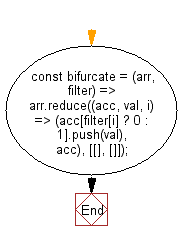# JavaScript: Split values of two given arrays into two groups

## JavaScript fundamental (ES6 Syntax): Exercise-17 with Solution

Write a JavaScript program to split values of two given arrays into two groups. If an element in filter is truthy, the corresponding element in the collection belongs to the first group; otherwise, it belongs to the second group.

• Use Array.prototype.reduce() and Array.prototype.push() to add elements to groups, based on filter.
• If filter has a truthy value for any element, add it to the first group, otherwise add it to the second group.

Sample Solution:

JavaScript Code:

``````//#Source https://bit.ly/2neWfJ2
const bifurcate = (arr, filter) =>
arr.reduce((acc, val, i) => (acc[filter[i] ? 0 : 1].push(val), acc), [[], []]);
console.log(bifurcate([1, 2, 3, 4], [true, true, false, true]));
console.log(bifurcate([1, 2, 3, 4], [true, true, true, true]));
console.log(bifurcate([1, 2, 3, 4], [false, false, false, false]));
```
```

Sample Output:

```[[1,2,4],]
[[1,2,3,4],[]]
[[],[1,2,3,4]]
```

Flowchart:Live Demo:

See the Pen javascript-basic-exercise-1-17 by w3resource (@w3resource) on CodePen.

Improve this sample solution and post your code through Disqus

What is the difficulty level of this exercise?

Test your Programming skills with w3resource's quiz.

﻿

## JavaScript: Tips of the Day

Chunks an array into n smaller arrays

Example:

```const tips_chunkIntoN = (arr, n) => {
const size = Math.ceil(arr.length / n);
return Array.from({ length: n }, (v, i) =>
arr.slice(i * size, i * size + size)
);
}
console.log(tips_chunkIntoN([1, 2, 3, 4, 5, 6, 7,8], 4));
```

Output:

```[[1,2],[3,4],[5,6],[7,8]]
```